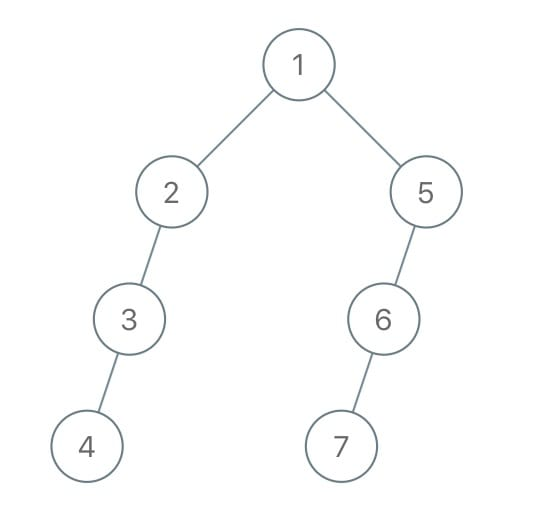# 1028. Recover a Tree From Preorder Traversal

Reference: LeetCode
Difficulty: Hard

## Problem

We run a preorder depth first search on the root of a binary tree.

At each node in this traversal, we output D dashes (where D is the depth of this node), then we output the value of this node. (If the depth of a node is D, the depth of its immediate child is D + 1. The depth of the root node is 0.)

If a node has only one child, that child is guaranteed to be the left child.

Given the output S of this traversal, recover the tree and return its root.

Example:## Analysis

Methods:

1. Recursion
• Do an iterative depth first search, parsing dashes from the string to know how to link the nodes together.
• Be careful of the corner cases.
• The value may consist of several digits.

Time: $O(S)$ where $S$ is the length of the string.
Space: $O(h)$

CommentJunhao Wang
a software engineering cat